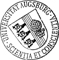## Value Function Iteration as a Solution Method for the Ramsey Model

• Value function iteration is one of the Standard tools for the solution of dynamic general equilibrium models if the dimension of the state space is one ore two. We consider three kinds of models: the deterministic and the stochastic growth model and a simple heterogenous agent model. Each model is solved with six different algorithms: (1) simple value function iteration as compared to (2) smart value function iteration neglects the special structure of the problem. (3) Full and (4) modified policy iteration are methods to speed up convergence. (5) linear and (6) cubic interpolation between the grid points are methods that enhance precision and reduce the size of the grid. We evaluate the algorithms with respect to speed and accuracy. Accuracy is defined as the maximum absolute value of the residual of the Euler equation that determines the household’s savings. We demonstrate that the run time of all algorithms can be reduced substantially if the value function is initialized stepwise,Value function iteration is one of the Standard tools for the solution of dynamic general equilibrium models if the dimension of the state space is one ore two. We consider three kinds of models: the deterministic and the stochastic growth model and a simple heterogenous agent model. Each model is solved with six different algorithms: (1) simple value function iteration as compared to (2) smart value function iteration neglects the special structure of the problem. (3) Full and (4) modified policy iteration are methods to speed up convergence. (5) linear and (6) cubic interpolation between the grid points are methods that enhance precision and reduce the size of the grid. We evaluate the algorithms with respect to speed and accuracy. Accuracy is defined as the maximum absolute value of the residual of the Euler equation that determines the household’s savings. We demonstrate that the run time of all algorithms can be reduced substantially if the value function is initialized stepwise, starting on a coarse grid and increasing the number of grid points successively until the desired size is reached. We find that value function iteration with cubic spline interpolation between grid points dominates the other methods if a high level of accuracy is needed.Author: Alfred MaußnerGND, Burkhard HeerGND urn:nbn:de:bvb:384-opus4-380535 https://opus.bibliothek.uni-augsburg.de/opus4/38053 0021-4027OPAC Journal of Economics and Statistics Jahrbücher für Nationalökonomie und Statistik Article English 2011 Universität Augsburg 2018/02/09 Value function iteration; policy function iteration; Howard's Algorithm; stochastic Ramsey model; acceleration; cubic interpolation; heterogeneous agents 231 4 494 515 Wirtschaftswissenschaftliche Fakultät Wirtschaftswissenschaftliche Fakultät / Institut für Volkswirtschaftslehre Wirtschaftswissenschaftliche Fakultät / Institut für Volkswirtschaftslehre / Lehrstuhl für Finanzwissenschaft Wirtschaftswissenschaftliche Fakultät / Institut für Volkswirtschaftslehre / Lehrstuhl für Empirische Makroökonomik 3 Sozialwissenschaften / 33 Wirtschaft / 330 WirtschaftDeutsches Urheberrecht